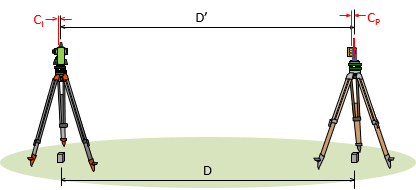## 1. Combined Constant

An EDM distance is the sum of the measured distance and the instrument and reflector constants, Figure E-1.Figure E-1Instrument and Reflector Constants

 D = D'+(CI+CP) Equation E-1 D: corrected distance D': measured distance         CI: instrument offset CR: reflector offsetk = (CI+CR)

k is the Combined Constant. Because it is internal, most instruments compensate for CI. The surveyor needs only to input the reflector constant; typical values are 0 mm and 30 mm. Over time the TSI may lose calibration and rough reflector handling require determination of a new constant to use.